# Cost Function - Theory of Production and Cost Notes | Study Business Economics for CA Foundation - CA Foundation

## CA Foundation: Cost Function - Theory of Production and Cost Notes | Study Business Economics for CA Foundation - CA Foundation

The document Cost Function - Theory of Production and Cost Notes | Study Business Economics for CA Foundation - CA Foundation is a part of the CA Foundation Course Business Economics for CA Foundation.
All you need of CA Foundation at this link: CA Foundation

COST FUNCTION
Cost function refers to the mathematical relation between cost of a product and the various determinants of costs. In a cost function, the dependent variable is unit cost or total cost and the independent variables are the price of a factor, the size of the output or any other relevant phenomenon which has a bearing on cost, such as technology, level of capacity utilization, efficiency and time period under consideration. Cost function is a function which is obtained from production function and the market supply of inputs. It expresses the relationship between costs and output. Cost functions are derived from actual cost data of the firms and are presented through cost curves. The shape of the cost curves depends upon the cost function. Cost functions are of two kinds: They are short-run cost functions and long-run cost functions.
SHORT RUN TOTAL COSTS
Total, fixed and variable costs: There are some factors which can be easily adjusted with changes in the level of output. A firm can readily employ more workers if it has to increase output. Similarly, it can purchase more raw materials if it has to expand production. Such factors which can be easily varied with a change in the level of output are called variable factors. On the other hand, there are some factors such as building, capital equipment, or top management team which cannot be so easily varied. It requires comparatively longer time to make changes in them. It takes time to install new machinery. Similarly, it takes time to build a new factory. Such factors which cannot be readily varied and require a longer period to adjust are called fixed factors.
Corresponding to the distinction between variable and fixed factors, we distinguish between short run and long run periods of time. Short run is a period of time in which output can be increased or decreased by changing only the amount of variable factors such as, labour, raw materials, etc. In the short run, quantities of fixed factors cannot be varied in accordance with changes in output. If the firm wants to increase output in the short run, it can do so only by increasing the variable factors, i.e., by using more labour and/or by buying more raw materials. Thus, short run is a period of time in which only variable factors can be varied, while the quantities of fixed factors remain unaltered. On the other hand, long run is a period of time in which the quantities of all factors may be varied. In other words, all factors become variable in the long run.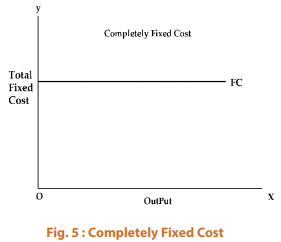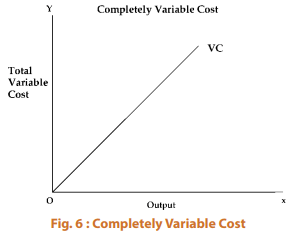Thus, we find that fixed costs are those costs which are independent of output, i.e., they do not change with changes in output. These costs are a “fixed amount” which are incurred by a firm in the short run, whether the output is small or large. Even if the firm closes down for some time in the short run but remains in business, these costs have to be borne by it. Fixed costs include such charges as contractual rent, insurance fee, maintenance cost, property taxes, interest on capital employed, managers’ salary, watchman’s wages etc. The fixed cost curve is presented in figure 5. Variable costs, on the other hand are those costs which change with changes in output. These costs include payments such as wages of casual labour employed, prices of raw material, fuel and power used, transportation cost etc. If a firm shuts down for a short period, it may not use the variable factors of production and therefore, will not therefore incur any variable cost. Figure 6 above presents completely variable cost curve drawn under the assumption that variable costs change linearly with changes in output.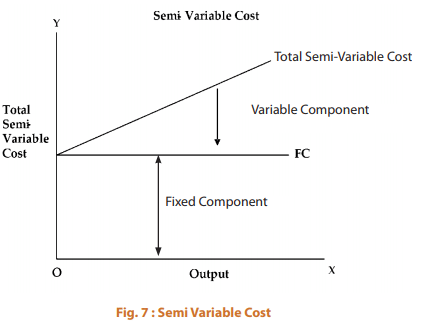There are some costs which are neither perfectly variable, nor absolutely fixed in relation to the changes in the size of output. They are known as semi-variable costs. Example: Electricity charges include both a fixed charge and a charge based on consumption.
There are some costs which may increase in a stair-step fashion, i.e., they remain fixed over certain range of output; but suddenly jump to a new higher level when output goes beyond a given limit. E.g. Costs incurred towards the salary of foremen will have a sudden jump if another foreman is appointed when the output crosses a particular limit.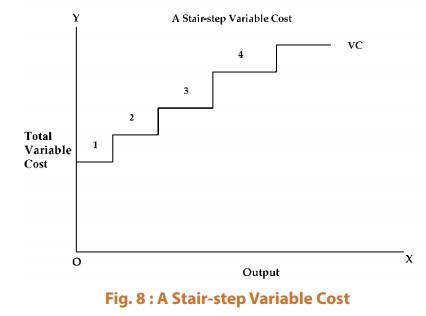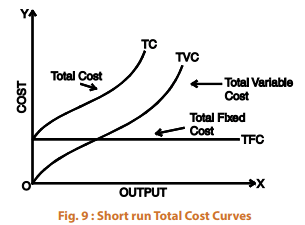The total cost of a business is defined as the actual cost that must be incurred for producing a given quantity of output. The short run total cost is composed of two major elements namely, total fixed cost and total variable cost. Symbolically TC = TFC + TVC. We may represent total cost, total variable cost and fixed cost diagrammatically.
In the diagram above, the total fixed cost curve (TFC) is a horizontal straight line parallel to X-axis as TFC remains fixed for the whole range of output. This curve starts from a point on the Y-axis meaning thereby that fixed costs will be incurred even if the output is zero. On the other hand, the total variable cost curve rises upward indicating that as output increases, total variable cost increases. The total variable cost curve starts from the origin because variable costs are zero when the output is zero. It should be noted that the total variable cost initially increases at a decreasing rate and then at an increasing rate with increases in output. This pattern of change in the TVC occurs due to the operation of the law of increasing and diminishing returns to the variable inputs. Due to the operation of diminishing returns, as output increases, larger quantities of variable inputs are required to produce the same quantity of output. Consequently, variable cost curve is steeper at higher levels of output. The total cost curve has been obtained by adding vertically the total fixed cost curve and the total variable cost curve. The slopes of TC and TVC are the same at every level of output and at each point the two curves have vertical distance equal to total fixed cost. Its position reflects the amount of fixed costs and its slope reflects variable costs.
Short run average costs
Average Fixed cost (AFC) : AFC is obtained by dividing the total fixed cost by the number of units of output produced. i.e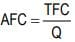where Q is the number of units produced. Thus, average fixed cost is the fixed cost per unit of output. For example, if a firm is producing with a total fixed cost of ₹ 2,000/-. When output is 100 units, the average fixed cost will be ₹ 20. And now, if the output increases to 200 units, average fixed cost will be ₹ 10. Since total fixed cost is a constant amount, average fixed cost will steadily fall as output increases. Therefore, if we draw an average fixed cost curve, it will slope downwards throughout its length but will not touch the X-axis as AFC cannot be zero. (Fig. 10)
Average variable cost (AVC) : Average variable cost is found out by dividing the total variable cost by the number of units of output produced, i.e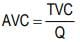where Q is the number of units produced. Thus, average variable cost is the variable cost per unit of output. Average variable cost normally falls as output increases from zero to normal capacity output due to occurrence of increasing returns to variable factors.
But beyond the normal capacity output, average variable cost will rise steeply because of the operation of diminishing returns (the concepts of increasing returns and diminishing returns have already been discussed earlier). If we draw an average variable cost curve, it will first fall, then reach a minimum and then rise. (Fig. 10)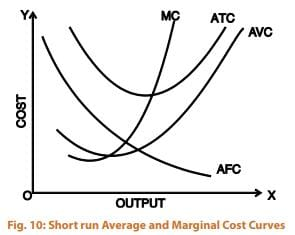Average total cost (ATC): Average total cost is the sum of average variable cost and average fixed cost. i.e., ATC = AFC + AVC. It is the total cost divided by the number of units produced, i.e. ATC = TC/Q.The behaviour of average total cost curve depends upon the behaviour of the average variable cost curve and the average fixed cost curve. In the beginning, both AVC and AFC curves fall, therefore, the ATC curve will also fall sharply. When AVC curve begins to rise, but AFC curve still falls steeply, ATC curve continues to fall. This is because, during this stage, the fall in AFC curve is greater than the rise in the AVC curve, but as output increases further, there is a sharp rise in AVC which more than offsets the fall in AFC. Therefore, ATC curve first falls, reaches its minimum and then rises. Thus, the average total cost curve is a “U” shaped curve. (Fig. 10)
Marginal cost: Marginal cost is the addition made to the total cost by the production of an additional unit of output. In other words, it is the total cost of producing t units instead of t-1 units, where t is any given number. For example, if we are producing 5 units at a cost of ₹ 200 and now suppose the 6th unit is produced and the total cost is ₹ 250, then the marginal cost is ₹ 250 - 200 i.e., ₹ 50. And marginal cost will be ₹ 24, if 10 units are produced at a total cost of ₹ 320 [(320-200) / (10-5)]. It is to be noted that marginal cost is independent of fixed cost. This is because fixed costs do not change with output. It is only the variable costs which change with a change in the level of output in the short run. Therefore, marginal cost is in fact due to the changes in variable costs. Symbolically marginal cost may be written as: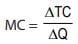DTC = Change in Total cost
DQ = Change in Output
or
MCn = TCn – TCn-1
Marginal cost curve falls as output increases in the beginning. It starts rising after a certain level of output. This happens because of the influence of the law of variable proportions. The MC curve becomes minimum corresponding to the point of inflexion on the total cost curve. The fact that marginal product rises first, reaches a maximum and then declines ensures that the marginal cost curve of a firm declines first, reaches its minimum and then rises. In other words marginal cost curve of a firm is “U” shaped (see Figure 10). The behaviour of these costs has also been shown in Table 2.

 Table 2 : Various Costs Units of Total Total Total Average Average Average Marginal output fixed cost variable cost cost fixed cost variable cost total cost cost 0 1000 0 1000 - - - - 1 1000 50 1050 1000.00 50.00 1050.00 50 2 1000 90 1090 500.00 45.00 545.00 40 3 1000 140 1140 333.33 46.67 380.00 50 4 1000 196 1196 250.00 49.00 299.00 56 5 1000 255 1255 200.00 51.00 251.00 59 6 1000 325 1325 166.67 54.17 220.83 70 7 1000 400 1400 142.86 57.14 200.00 75 8 1000 480 1480 125.00 60.00 185.00 80 9 1000 570 1570 111.11 63.33 174.44 90 10 1000 670 1670 100.00 67.00 167.00 100 11 1000 780 1780 90.91 70.91 161.82 110 12 1000 1080 2080 83.33 90.00 173.33 300

The above table shows that:
(i) Fixed costs do not change with increase in output upto a given level. Average fixed cost, therefore, comes down with every increase in output.
(ii) Variable costs increase, but not necessarily in the same proportion as the increase in output. In the above case, average variable cost comes down gradually till 4 units are produced. Thereafter it starts increasing.
(iii) Marginal cost is the additional cost divided by the additional units produced. This also comes down first and then starts increasing.
Relationship between Average Cost and Marginal Cost: The relationship between marginal cost and average cost is the same as that between any other marginal-average quantities. The following are the points of relationship between the two.
(1) When average cost falls as a result of an increase in output, marginal cost is less than average cost.
(2) When average cost rises as a result of an increase in output, marginal cost is more than average cost.
(3) When average cost is minimum, marginal cost is equal to the average cost. In other words, marginal cost curve cuts average cost curve at its minimum point (i.e. optimum point).
Figure 10 confirms the above points of relationship

LONG RUN AVERAGE COST CURVE
As stated above, long run is a period of time during which the firm can vary all of its inputs; unlike short run in which some inputs are fixed and others are variable. In other words, whereas in the short run the firm is tied with a given plant, in the long run the firm can build any size or scale of plant and therefore, can move from one plant to another; it can acquire a big plant if it wants to increase its output and a small plant if it wants to reduce its output. The long run being a planning horizon, the firm plans ahead to build the most appropriate scale of plant to produce the future level of output. It should be kept in mind that once the firm has built a particular scale of plant, its production takes place in the short run. Briefly put, the firm actually operates in the short run and plans for the long run. Long run cost of production is the least possible cost of producing any given level of output when all individual factors are variable. A long run cost curve depicts the functional relationship between output and the long run cost of production.
In order to understand how the long run average cost curve is derived, we consider three short run average cost curves as shown in Figure 11. These short run average cost curves (SACs) are also called ‘plant curves’. In the short run, the firm can be operating on any short run average cost curve, given the size of the plant. Suppose that there are the only three plants which are technically possible. Given the size of the plant, the firm will be increasing or decreasing its output by changing the amount of the variable inputs. But in the long run, the firm chooses among the three possible sizes of plants as depicted by short run average curves (SAC1 , SAC2 , and SAC3 ). In the long run, the firm will examine with which size of plant or on which short run average cost curve it should operate to produce a given level of output, so that the total cost is minimum. It will be seen from the diagram that up to OB amount of output, the firm will operate on the SAC1 , though it could also produce with SAC2 . Up to OB amount of output, the production on SAC1 results in lower cost than on SAC2 . For example, if the level of output OA is produced with SAC1 , it will cost AL per unit and if it is produced with SAC2 it will cost AH and we can see that AH is more than AL. Similarly, if the firm plans to produce an output which is larger than OB but less than OD, then it will not be economical to produce on SAC1 . For this, the firm will have to use SAC2 . Similarly, the firm will use SAC3 for output larger than OD. It is thus clear that, in the long run, the firm has a choice in the employment of plant and it will employ that plant which yields minimum possible unit cost for producing a given output.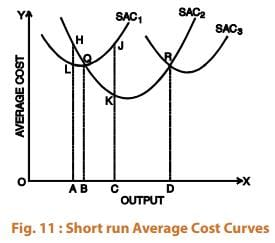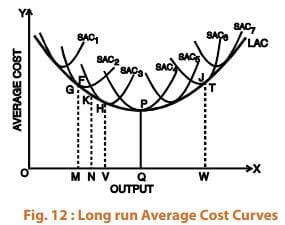Suppose, the firm has a choice so that a plant can be varied by infinitely small gradations so that there are infinite number of plants corresponding to which there are numerous average cost curves. In such a case the long run average cost curve will be a smooth curve enveloping all these short run average cost curves.
As shown in Figure 12, the long run average cost curve is so drawn as to be tangent to each of the short run average cost curves. Every point on the long run average cost curve will be a tangency point with some short run AC curve. If a firm desires to produce any particular output, it then builds a corresponding plant and operates on the corresponding short run average cost curve. As shown in the figure, for producing OM, the corresponding point on the LAC curve is G and the short run average cost curve SAC2 is tangent to the long run AC at this point. Thus, if a firm desires to produce output OM, the firm will construct a plant corresponding to SAC2 and will operate on this curve at point G. Similarly, the firm will produce other levels of output choosing the plant which suits its requirements of lowest possible cost of production. It is clear from the figure that larger output can be produced at the lowest cost with larger plant whereas smaller output can be produced at the lowest cost with smaller plants. For example, to produce OM, the firm will be using SAC2 only; if it uses SAC3 , it will result in higher unit cost than SAC2 . But, larger output OV can be produced most economically with a larger plant represented by the SAC3 . If we produce OV with a smaller plant, it will result in higher cost per unit. Similarly, if we produce larger output with a smaller plant it will involve higher costs because of its limited capacity
It is to be noted that LAC curve is not tangent to the minimum points of the SAC curves. When the LAC curve is declining, it is tangent to the falling portions of the short run cost curves and when the LAC curve is rising, it is tangent to the rising portions of the short run cost curves. Thus, for producing output less than “OQ” at the lowest possible unit cost, the firm will construct the relevant plant and operate it at less than its full capacity, i.e., at less than its minimum average cost of production. On the other hand, for outputs larger than OQ the firm will construct a plant and operate it beyond its optimum capacity. “OQ” is the optimum output. This is because “OQ” is being produced at the minimum point of LAC and corresponding SAC i.e., SAC4 . Other plants are either used at less than their full capacity or more than their full capacity. Only SAC4 is being operated at the minimum point.
The long run average cost curve is often called as ‘planning curve’ because a firm plans to produce any output in the long run by choosing a plant on the long run average cost curve corresponding to the given output. The long run average cost curve helps the firm in the choice of the size of the plant for producing a specific output at the least possible cost.

Explanation of the “U” shape of the long run average cost curve: As has been seen in the diagram LAC curve is a “U” shaped curve. This shape of LAC curve has nothing to do with the U shaped SAC which is due to variable factor ratio because in the long run all factors are variable. U shaped LAC arises due to returns to scale. As discussed earlier, when the firm expands, returns to scale increase. After a range of constant returns to scale, the returns to scale finally decrease. On the same line, the LAC curve first declines and then finally rises. Increasing returns to scale cause fall in the long run average cost and decreasing returns to scale result in rise in long run average cost. Falling long run average cost and increasing economies of scale result from internal and external economies of scale and rising long run average cost and diminishing returns to scale result from internal and external diseconomies of scale. (Economies of scale will be discussed in the next section.)
The long run average cost curve initially falls with increase in output and after a certain point it rises making a boat shape. The long-run average cost (LAC) curve is also called the planning curve of the firm as it helps in choosing an appropriate a plant on the decided level of output. The long-run average cost curve is also called “Envelope curve”, because it envelopes or supports a family of short run average cost curves from below.
The above figure depicting long-run average cost curve is arrived at on the basis of traditional economic analysis. It is flattened ‘U’ shaped. This type of curve could exist only when the state of technology remains constant. But, empirical evidence shows modern firms face ‘L-shaped’ cost curve over a considerable quantity of output. The L-shaped long run cost curve implies that initially when the output is increased due to increase in the size of plant (and associated variable factors), per unit cost falls rapidly due to economies of scale. The long-run average cost curve does not increase even after a sufficiently large scale of output as it continues to enjoy economies of scale.

The document Cost Function - Theory of Production and Cost Notes | Study Business Economics for CA Foundation - CA Foundation is a part of the CA Foundation Course Business Economics for CA Foundation.
All you need of CA Foundation at this link: CA FoundationUse Code STAYHOME200 and get INR 200 additional OFF

## Business Economics for CA Foundation

61 videos|111 docs|67 tests

Track your progress, build streaks, highlight & save important lessons and more!

,

,

,

,

,

,

,

,

,

,

,

,

,

,

,

,

,

,

,

,

,

;